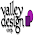## Mar 5, 2018

### Silicon carbide of Ni/6H-SiC and Ti/4H-SiC type Schottky diode current-voltage characteristics modelling

On the base of the physical analytical models based on Poisson's equation, drift–diffusion and continuity equations the forward current–voltage characteristics of 6H-SiC and 4H-SiC type Schottky diode with Ni and Ti Schottky contact have been simulated. It is shown on the base of analysis of current–voltage characteristics in terms of classical thermionic emission theory it is shown that the proposed simulation model of Schottky diode corresponds to the almost "ideal" diode with ideality factor n equals 1.1. Because of this it is determined that the effective Schottky barrier heightB equals 1.57 eV and 1.17 eV for Ni/6H and Ti/4H silicon carbide Schottky diode type, respectively.

Source:IOPscience

1.2.